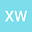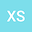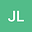Application of a Flood Risk Assessment Model Combining Analytic Hierarchy Process Modified by Trapezoidal Fuzzy Number and Variable Fuzzy Set Theory: A Case Study in Zhejiang Province, China.
•••• +1
• Xu Wu,
• Xiaojing Shen,
• Jianshe Li,
• Meijian Yang
Xu Wu
Ningxia University

Corresponding Author:[email protected]

Author ProfileXiaojing Shen
Ningxia University
Author ProfileJianshe Li
Ningxia University
Author ProfileMeijian Yang
University of Connecticut
Author Profile## Abstract

Abstract: The scientific evaluation of regional flood risk is particularly important for flood management. Past studies build a hierarchy evaluation index system with two factors and ten sub-factors to assess flood hazard risk, each factor contains five sub-factors. The weights of the sub-factors in their respective evaluation systems are (0.065, 0.129, 0.210, 0.215, 0.378) and (0.399, 0.152, 0.155, 0.180, 0.109) respectively, from expert scoring results optimized by Analytic Hierarchy Process (AHP) modified by Trapezoidal Fuzzy Number (TFN). Variable Fuzzy Set Theory (VFST) is adopted to calculate the risk values of the two factors, the weighted average of two factors risk values is used as flood disaster risk value. Based on above steps, a flood disaster evaluation model is established and applied to evaluate regional flood risks in Zhejiang Province. Extremely low risk, low risk, moderate risk, high risk, extremely high risk areas are 51017 km2,10090 km2,10112 km2,13428 km2 and 19153 km2, respectively occupy with 49.15%, 9.72%, 9.74%, 12.94%, and 18.45% of study region. TFN-modified AHP calculates the weights of sub-factors, considering the subjective cognition of experts and the objective response of factors, which makes the results more representative and scientific. Application of VFST decreases the randomness and uncertainty of flood risk assessment, which improves the stability of evaluation process and reliability of evaluation results. Meanwhile, 30×30m basic evaluation unit adopted in this study accurately and objectively represent the spatial distribution of flood hazard in Zhejiang Province, China. The evaluation results of the model are validated to be consistent with the actual situation of flood disaster.